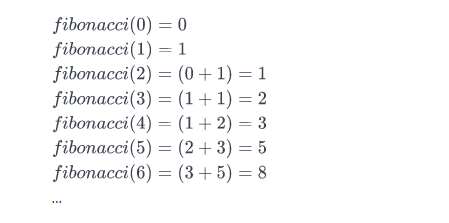## Consume php backend nested array on a C# Application – Json.NET

Lets assume you got a MYSQL database that contains data for your application and you want to consume this information on a C# Application in order to handle it on a client.

For the sake of this example the following table will be parsed

As depicted the following information is returned from the api:

• the state of the response (succeeded or not)
• a message that will show the response result
• the actual result that in this case is a list of trips

Each trip contains id, fromlocation, tolocation, date and time properties that have to be returned to the application.

The php code that was used to create the data from the database is the following:

So in order to parse this json result we will need a plugin like Newtonsoft.Json

The class that will be used to encapsulate the response would be the following:

And then we will consume the json response with the following code in C#. In this example data from the API came from a POST request on a web Form.

## Send signals between Mainpage.xaml.cs and Viewmodel – Xamarin.Forms

In a recent application I wanted to inform a Listview with a specific ItemTemplate to update its data source on back button with a synchronous data fetching mechanism(so this was not automatic).

So the goal was to send a “signal” to my Viewmodel when the page was loaded to perform an action. This can be done using MessageCenter with Xamarin.Forms.

The solution was to send an update signal every time OnAppearing() method of the MainPage.xaml was hit and inform the ViewModel to perfom an action.

Then perform action on MainPageViewModel

## Find the Nth Fibonacci Number – C# Code

The Fibonacci sequence begins with Fibonacci(0) = 0  and Fibonacci(1)=1  as its respective first and second terms. After these first two elements, each subsequent element is equal to the sum of the previous two elements.

Here is the basic information you need to calculate Fibonacci(n):

• Fibonacci(0) = 0
• Fibonacci(1) = 1
• Fibonacci(n) = Fibonacci(n-1) + Fibonacci(n-2)

Given n, complete the fibonacci function so it returns Fibonacci(n).

Input Format

Nth number

Output Format

Fibonacci() Nth number.

Sample Input

Sample Output

Explanation

Consider the Fibonacci sequence:We want to know the value of Fibonacci(3). If we look at the sequence above,Fibonacci(3)  evaluates to 2. Thus, we print 2 as our answer.